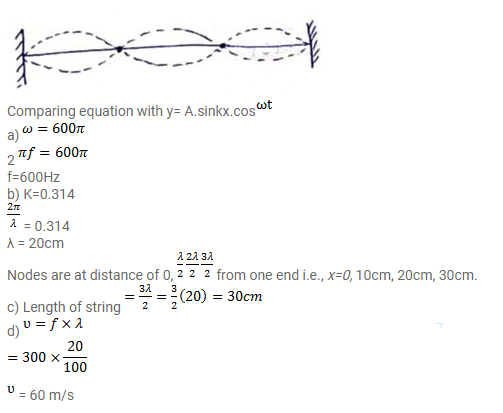# The equation for the vibration of a string,Question:

The equation for the vibration of a string, fixed at both ends vibrating in its third harmonic is given by $\mathrm{y}=(0.4 \mathrm{~cm}) \sin \left[\left(0.314 \mathrm{~cm}^{-1}\right) \mathrm{x}\right] \cos \left[\left(600 \pi \mathrm{s}^{-1}\right) \mathrm{t}\right]$

a. At what frequency of vibration?

b. What are the position of the nodes?

c. What is the length of the string?

d. What is the wavelength and the speed of two travelling waves that can interfere to give this vibration?

Solution: